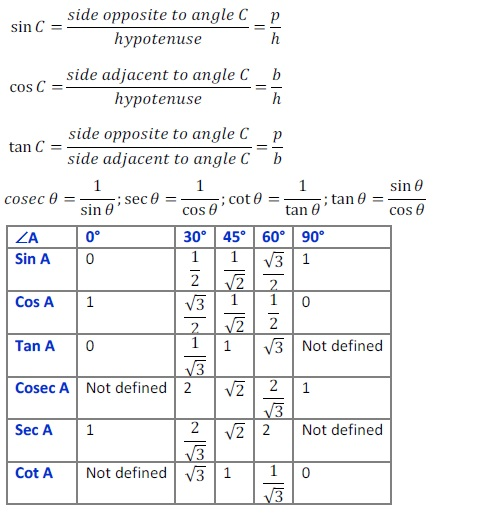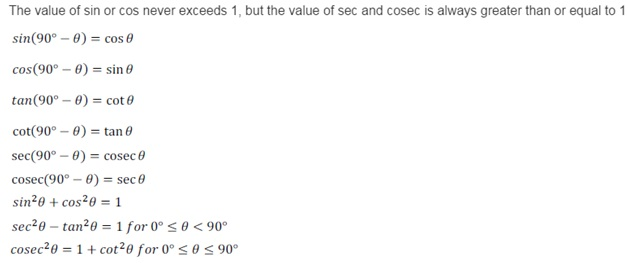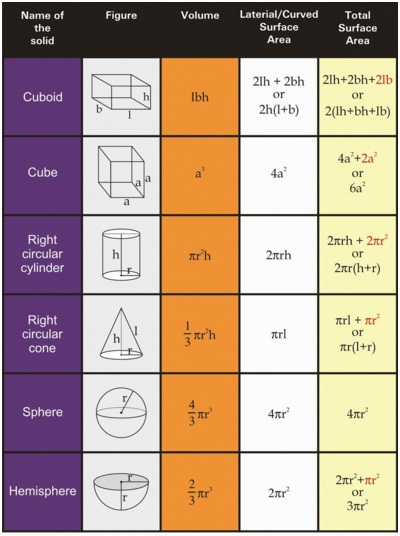# Important Maths Formulae for Class 10 CBSE

Most of the students are math-phobic; they think it’s an extremely tough subject that they can’t master at all. This negative attitude towards their subject stops them from focusing on math problems. They get nervous before exams, even before a small class test, as they are not at all prepared. To get rid of this situation, students need to hold a strong grip on this subject. If you hold it tightly, you can collect as many marks as you want. Understand the basic concepts, learn all the important formulae, apply formulae according to the concept and crack the most difficult question.

IMPORTANT MATHS FORMULAE

Algebra:

1. (a + b)2 = a2 + 2ab + b2
1. (a – b)2  = a2 – 2ab + b2
1. (a + b) (a – b) = a2 – b2
1. (x + a)(x + b)  = x2 + (a + b)x + ab
1. (x + a)(x – b)  = x2 + (a – b)x – ab
1. (x – a)(x + b)  = x2 + (b – a)x – ab
1. (x – a)(x – b)  = x2  – (a + b)x + ab
1. (a + b)3  = a3 + b3 + 3ab(a + b)
1. (a – b)3  =  a3 – b3 – 3ab(a – b)
1. (x + y + z) 2  = x2 + y2 + z2 + 2xy + 2yz + 2xz
1. (x + y – z) 2  =  x2 + y2 + z2 + 2xy – 2yz – 2xz
1. (x – y + z)2  = x2 + y2 + z2 – 2xy – 2yz + 2xz
1. (x – y – z)2  = x2 + y2 + z2 – 2xy +  2yz – 2xz
1. x3 + y3 + z3 – 3xyz  = (x + y + z)(x2 + y2 + z2 – xy – yz -xz)
1. x2 + y2  = 1212 [(x + y)2 + (x – y)2]
1. (x + a) (x + b) (x + c) = x3 + (a + b +c)x2 + (ab + bc + ca)x + abc
1. x3 + y3 = (x + y) (x2 – xy + y2)
1. x3 – y3  = (x – y) (x2 + xy + y2)
1. x2 + y2 + z2 -xy – yz – zx = 1212 [(x-y)2 + (y-z)2 + (z-x)2]

Powers:

amxan  = am+n

aman=am−naman=am−n

(am)n  =  amn

(ambn)p =   ampb np

a-m = 1am1am

amn=am−−−√namn=amn

Rules of Zero:

a1  = a

a0 = 1

a*0 = 0

a is undefined

Linear Equation:

Linear equation in one variable ax + b = 0, x = – −ba−ba

Quadratic Equation:  ax2 + bx + c  = 0  x  = −b±b2−4ac√2a−b±b2−4ac2a

Discriminant D = b2 – 4ac

Math Formulas:

When the rate of discount is given, Discount =   MP∗Rate of Discount 100

Simple Interest = PTR100PTR100 where P = Principal, T = Time in years R = Rate of interest per annum

Principal = 100∗S.IR∗T100∗S.IR∗T

Rate = 100∗S.IP∗T100∗S.IP∗T

Time = 100∗S.IP∗R100∗S.IP∗R

Principal = Amount – Simple Interest

Discount = MP – SP

Trigonometry:Probability

P(E) =   Number of outcomes favourable to E

Total Number of Possible Outcomes

Mensuration